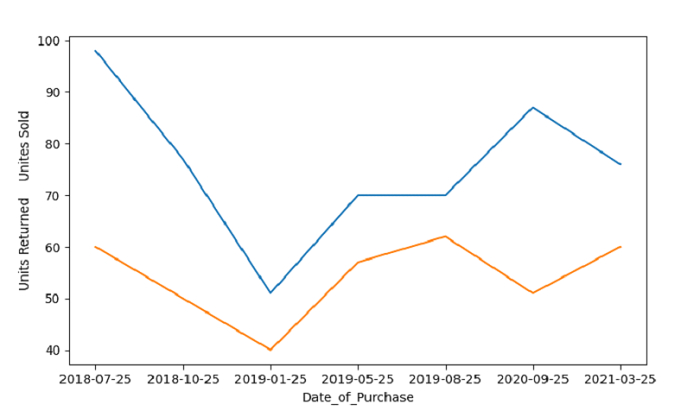# Python - Create a Time Series Plot with multiple columns using Line Plot

To create a Time Series Plot with multiple columns using Line Plot, use the lineplot(). At first, import the required libraries −

import seaborn as sb
import pandas as pd
import matplotlib.pyplot as plt

Create a DataFrame. We have multiple columns in our DataFrame −

dataFrame = pd.DataFrame({'Date_of_Purchase': ['2018-07-25', '2018-10-25', '2019-01-25', '2019-05-25', '2019-08-25','2020-09-25','2021-03-25'],'Units Sold': [98, 77, 51, 70, 70, 87, 76],'Units Returned' : [60, 50, 40, 57, 62, 51, 60]
})

Plot time series plot for multiple columns −

sb.lineplot(x="Date_of_Purchase", y="Units Sold", data=dataFrame)
sb.lineplot(x="Date_of_Purchase", y="Units Returned", data=dataFrame)

## Example

Following is the code −

import seaborn as sb
import pandas as pd
import matplotlib.pyplot as plt

# creating DataFrame
dataFrame = pd.DataFrame({'Date_of_Purchase': ['2018-07-25', '2018-10-25', '2019-01-25', '2019-05-25', '2019-08-25','2020-09-25','2021-03-25'],'Units Sold': [98, 77, 51, 70, 70, 87, 76],'Units Returned' : [60, 50, 40, 57, 62, 51, 60]
})

# time series plot for multiple columns
sb.lineplot(x="Date_of_Purchase", y="Units Sold", data=dataFrame)
sb.lineplot(x="Date_of_Purchase", y="Units Returned", data=dataFrame)

# set label
plt.ylabel("Units Returned Unites Sold")

plt.show()

## Output

This will produce the following output −